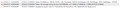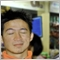# OrderSend Error 131, Although free margin is available.28

hey everyone, so I'm new to forex and also mt4. I am trying to build up a trading bot but when I try to place orders It gives me an ' not enough free margin error '.

So here is my code:

```#define MAGICNUM  20131111

// Define our Parameters
input double Lots          = 0.1;
input int PeriodOne        = 40; // The period for the first SMA
input int PeriodTwo        = 100; // The period for the second SMA
input int TakeProfit       = 40; // The take profit level (0 disable)
input int StopLoss         = 0; // The default stop loss (0 disable)
//+------------------------------------------------------------------+
//| expert initialization functions                                  |
//+------------------------------------------------------------------+
int init()
{
return(0);
}
int deinit()
{
return(0);
}
//+------------------------------------------------------------------+
//| Check for cross over of SMA                                      |
//+------------------------------------------------------------------+
int CheckForCross(double input1, double input2)
{
static int previous_direction = 0;
static int current_direction  = 0;

// Up Direction = 1
if(input1 > input2){
current_direction = 1;
}

// Down Direction = 2
if(input1 < input2){
current_direction = 2;
}

// Detect a direction change
if(current_direction != previous_direction){
previous_direction = current_direction;
return (previous_direction);
} else {
return (0);
}
}

//+------------------------------------------------------------------+
//| Calculate optimal lot size                                       |
//+------------------------------------------------------------------+
double LotsOptimized()
{
double lot = Lots;
// Calculate Lot size as a fifth of available free equity.
lot = NormalizeDouble((AccountFreeMargin()/
5)/1000.0,1);
if(lot<0.1) lot=0.1; //Ensure the minimal amount is 0.1 lots
return(lot);
}

//+------------------------------------------------------------------+
//+ Break Even                                                       |
//+------------------------------------------------------------------+
bool BreakEven(int MN){
int Ticket;

for(int i = OrdersTotal() - 1; i >= 0; i--) {

if(OrderSymbol() == Symbol() && OrderMagicNumber() == MN){
Ticket = OrderModify(OrderTicket(), OrderOpenPrice(), OrderOpenPrice(), OrderTakeProfit(), 0, Green);
if(Ticket < 0) Print("Error in Break Even : ", GetLastError());
break;
}
}

return(Ticket);
}

//+------------------------------------------------------------------+
//+ Run the algorithm                                               |
//+------------------------------------------------------------------+
int start()
{
int cnt, ticket, total;
double shortSma, longSma, ShortSL, ShortTP, LongSL, LongTP;

// Parameter Sanity checking
if(PeriodTwo < PeriodOne){
Print("Please check settings, Period Two is lesser then the first period");
return(0);
}

if(Bars < PeriodTwo){
Print("Please check settings, less then the second period bars available for the long SMA");
return(0);
}

// Calculate the SMAs from the iMA indicator in MODE_SMMA using the close price
shortSma = iMA(NULL, 0, PeriodOne, 0, MODE_SMMA, PRICE_CLOSE, 0);
longSma = iMA(NULL, 0, PeriodTwo, 0, MODE_SMMA, PRICE_CLOSE, 0);

// Check if there has been a cross on this tick from the two SMAs
int isCrossed = CheckForCross(shortSma, longSma);

// Get the current total orders
total = OrdersTotal();

// Calculate Stop Loss and Take profit
if(StopLoss > 0){
ShortSL = Bid+(StopLoss*Point);
}
if(TakeProfit > 0){
ShortTP = Bid-(TakeProfit*Point);
}

// Only open one trade at a time..
if(total < 1){
if(isCrossed == 1){
if(ticket > 0){
Print("BUY Order Opened: ", OrderOpenPrice(), " SL:", LongSL, " TP: ", LongTP);
}
else
Print("Error Opening BUY  Order: ", GetLastError());
return(0);
}
// Sell - Short position
if(isCrossed == 2){
ticket = OrderSend(Symbol(), OP_SELL, LotsOptimized(),Bid,5, ShortSL, ShortTP, "Double SMA Crossover",MAGICNUM,0,Red);
if(ticket > 0){
Print("SELL Order Opened: ", OrderOpenPrice(), " SL:", ShortSL, " TP: ", ShortTP);
}
else
Print("Error Opening SELL Order: ", GetLastError());
return(0);
}
}

// Manage open orders for exit criteria
for(cnt = 0; cnt < total; cnt++){
if(OrderType() <= OP_SELL && OrderSymbol() == Symbol()){
// Look for long positions
// Check for Exit criteria on buy - change of direction
if(isCrossed == 2){
OrderClose(OrderTicket(), OrderLots(), Bid, 3, Violet); // Close the position
return(0);
}
}
else //Look for short positions - inverse of prior conditions
{
// Check for Exit criteria on sell - change of direction
if(isCrossed == 1){
OrderClose(OrderTicket(), OrderLots(), Ask, 3, Violet); // Close the position
return(0);
}
}
// If we are in a loss - Try to BreakEven
Print("Current Unrealized Profit on Order: ", OrderProfit());
if(OrderProfit() < 0){
BreakEven(MAGICNUM);
}
}

}

return(0);
}```

When I try to run it with initial 1000\$ deposit, on EUR/USD parity. It doesn't work and give the error :errr
• ibb.co
Image errr hosted in imgbb.com2176

well, try this..

```bool MarginCheck (int TypeOfOrder, double LotValue){
double MarginValue = AccountFreeMarginCheck(Symbol(),TypeOfOrder,LotValue);
if(MarginValue <= 0){
return false;
}
return true;
}

//then

}
//or
if(MarginCheck(OP_SELL, Lots)==true){
}
```

trade will only open when there is enough margin..

hope this help.. goodluck..20207

If you are worried about margin and leverage, you are not controlling your risk. Never risk more than a small percentage of your account, certainly less than 2% per trade, 6% total.
1. In code (MT4): Risk depends on your initial stop loss, lot size, and the value of the pair. It does not depend on margin and leverage.
1. You place the stop where it needs to be - where the reason for the trade is no longer valid. E.g. trading a support bounce the stop goes below the support.
2. AccountBalance * percent/100 = RISK = OrderLots * (|OrderOpenPrice - OrderStopLoss| * DeltaPerLot + CommissionPerLot) (Note OOP-OSL includes the spread, and DeltaPerLot is usually around \$10/pip but it takes account of the exchange rates of the pair vs. your account currency.)
3. Do NOT use TickValue by itself - DeltaPerLot and verify that MODE_TICKVALUE is returning a value in your deposit currency, as promised by the documentation, or whether it is returning a value in the instrument's base currency.
MODE_TICKVALUE is not reliable on non-fx instruments with many brokers - MQL4 programming forum 2017.10.10
Lot value calculation off by a factor of 100 - MQL5 programming forum 2019.07.19
4. You must normalize lots properly and check against min and max.
5. You must also check FreeMargin to avoid stop out

Most pairs are worth about \$10 per PIP. A \$5 risk with a (very small) 5 PIP SL is \$5/\$10/5 or 0.1 Lots maximum.

2. Use a GUI EA like mine (for MT4): 'Money Manager Graphic Tool' indicator by 'takycard' - Risk Management - Articles, Library comments - MQL5 programming forum - Page 6 #55